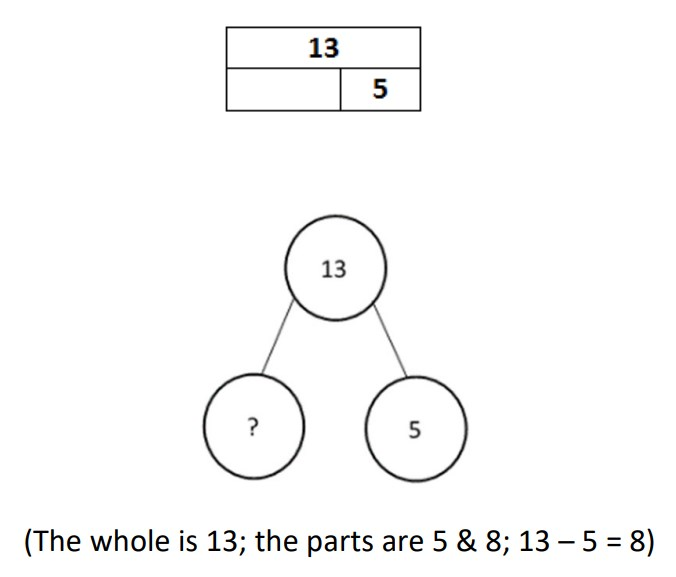# 2nd Grade, Activity 13: Represent and Solve Problems Involving Addition and Subtraction

Use addition and subtraction within 100 to solve one-and two-step word problems involving situations of adding to, taking from, putting together, taking apart, and comparing, with unknowns in all positions, e.g., by using drawings and equations with a symbol for the unknown number to represent the problem(Addition and Subtraction Problems Solving(Addition and Subtraction Problems Solving).

### Part-Part-Whole

• Group: Whole Class
• Materials: Part-Part-Whole sheet, paper and pencil

Explain that students will use a bar diagram and number bonds to organize the information in word problems to identify the part-part-whole. Explain that students will be finding either a part or whole in a problem. Write the problem below on the board. Help students identify the parts and whole in the problem and which one they are trying to find.

Sally put some pictures in a book. Only 13 of them were good. She gave 5 of them to friends. How many pictures does she have left?Distribute the Part-Part-Whole sheet to each student. Guide students through some of the problems to help them determine where in each diagram to write the numbers. Use part-whole language as each problem is reviewed. Have students write appropriate number sentences to match the diagrams. After an allotted time, invite three volunteers at a time to model how they displayed the problems using bar diagrams, number bonds, and number sentences.